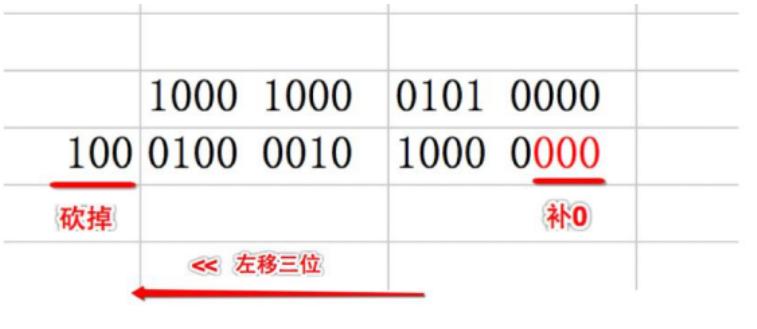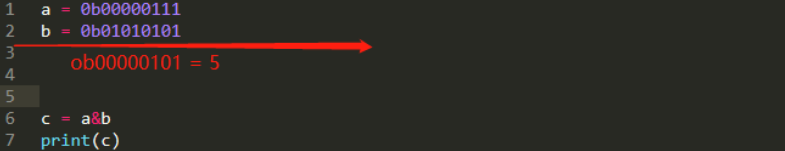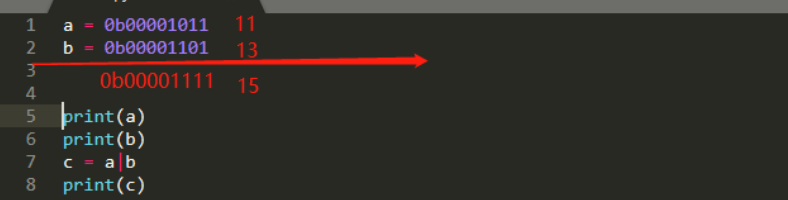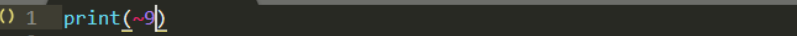Python中的位运算符有哪些Python中的位运算符有哪些？

1.位运算的介绍

& 按位与

| 按位或

^ 按位异或

~ 按位取反

<< 按位左移

> 按位右移

2.位运算

1）<< 按位左移x << n 左移 x 的所有二进制位向左移动n位,移出位删掉,移进的位补零

num = 0b00000001
print(num)
num = num<<1
print(num)
num = num<<1
print(num)
num = num<<1
print(num)
num = num<<1
print(num)

1
2
4
8
16

【注意事项】

2)>> 右移

x >> n x的所有二进制位向右移动n位,移出的位删掉,进的位补符号位 右移不会改变一个数的符号

【注意事项】

x 右移 n 位就相当于除以2的n次方 用途:快速计算一个数除以2的n次方 (8>>3 等同于8/2^3)

num = 0b10000000
print(num)
num = num>>1
print(num)
num = num>>1
print(num)
num = num>>1
print(num)
num = num>>1
print(num)

128
64
32
16
8

3)& 按位与4) | 按位或5) ^ 按位异或6) ~ 取反【为什么9取反变成了-10的说明】：

9的原码 ==> 0000 1001 因为正数的原码=反码=补码，所以在 真正存储的时

Python开发开发项目